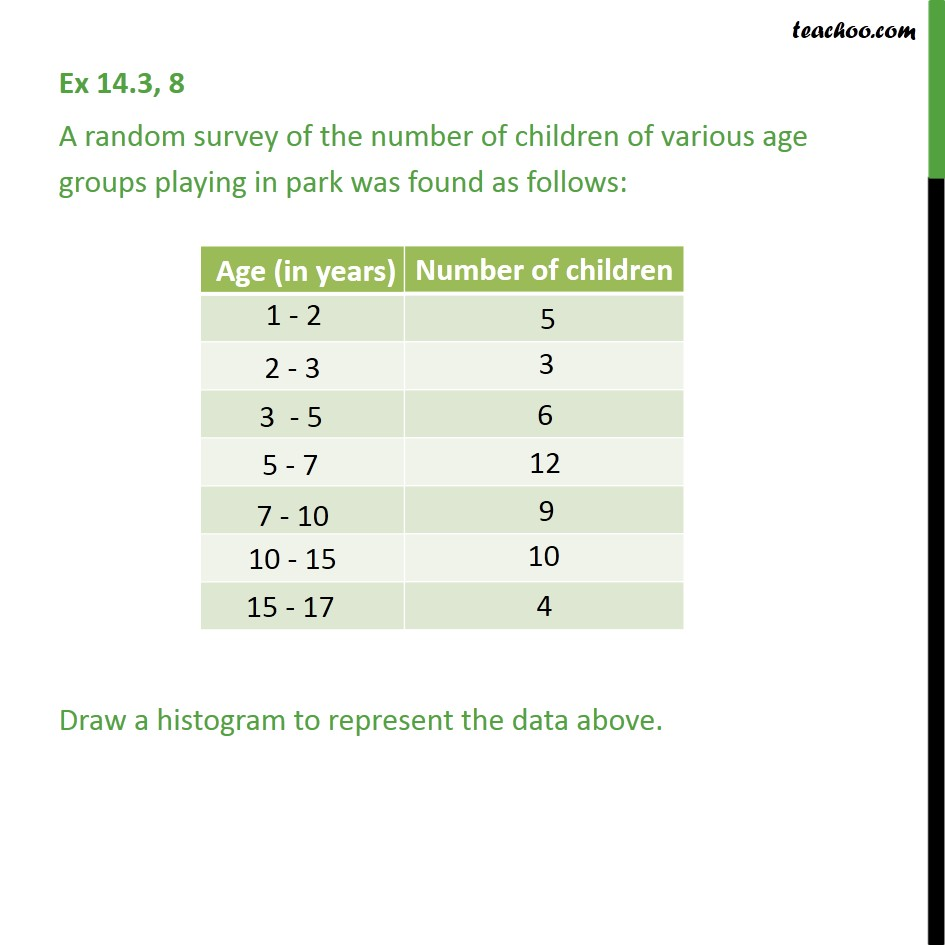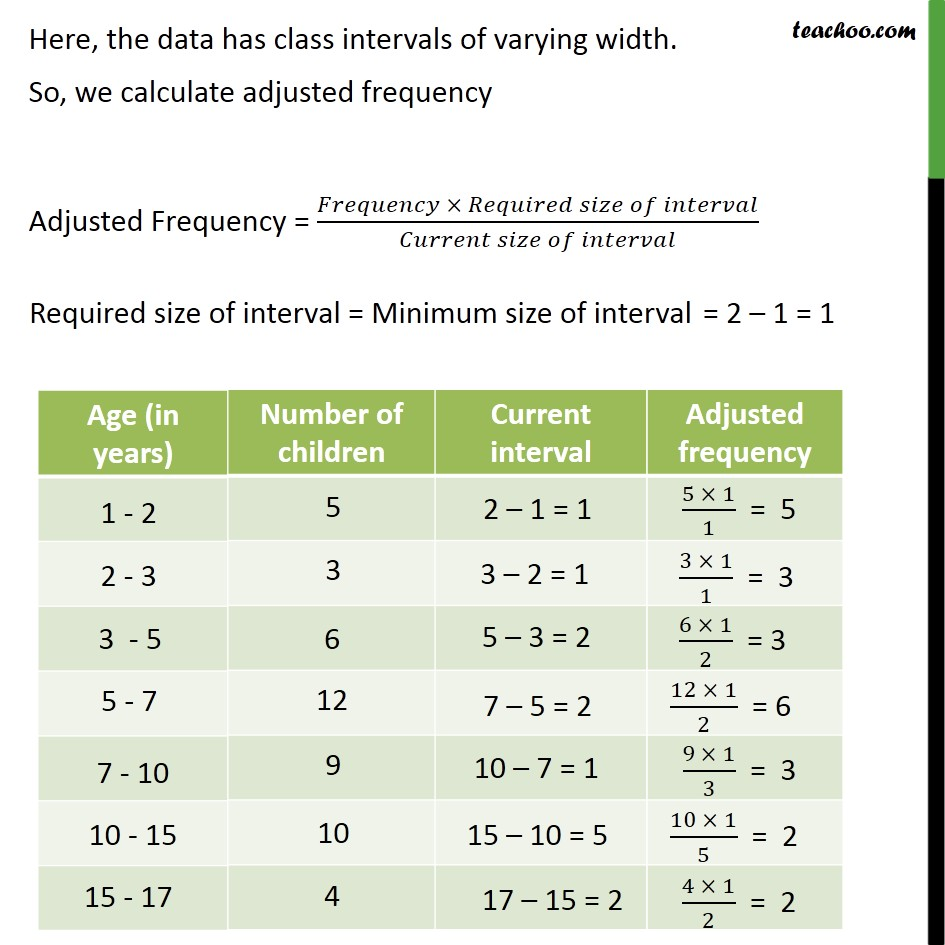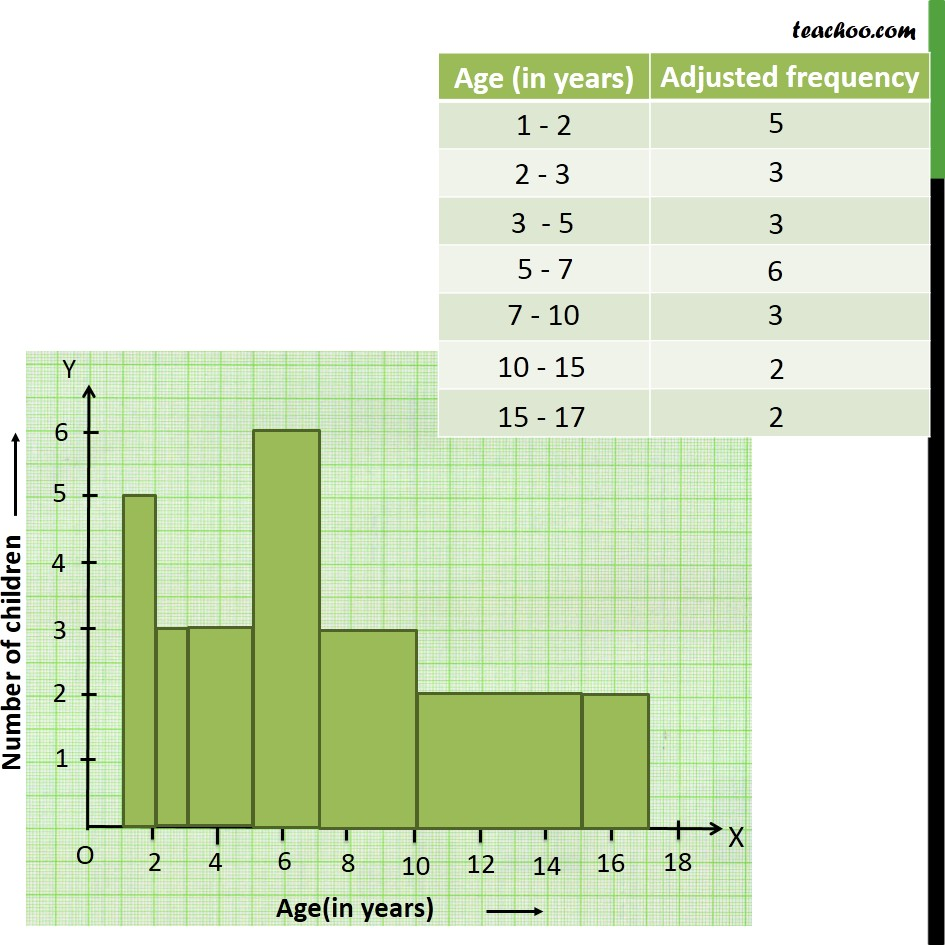1. Chapter 14 Class 9 Statistics
2. Serial order wise
3. Ex 14.3

Transcript

Ex 14.3, 8 A random survey of the number of children of various age groups playing in park was found as follows: Draw a histogram to represent the data above. Here, the data has class intervals of varying width. So, we calculate adjusted frequency Adjusted Frequency = ( )/( )

Ex 14.3

Chapter 14 Class 9 Statistics
Serial order wise